Select Page

In a previous couple of articles, we explored some basic machine learning algorithms. Thus far we covered some simple regression algorithms, classification algorithms and we started with algorithms that can be used for both types of problems, like SVM and Decision Trees. We used technologies like TensorFlow, Pytorch and SciKit Learn for the implementation and application of these algorithms. Apart from that, we used optimization techniques such as Gradient Descent.

Up to this point, we explored algorithms that are using supervised learning. This means that we always had input and expected output data that we used to train our machine learning models. In this type of learning, the training set contains inputs and desired outputs. This way the algorithm can check its calculated output the same as the desired output and take appropriate actions based on that.

Are you afraid that AI might take your job? Make sure you are the one who is building it.

STAY RELEVANT IN THE RISING AI INDUSTRY! 🖖

However, in real life, we often don’t have both input and output data, but we only have input data. This means that the algorithm on itself needs to figure connections between input samples. For that, we use unsupervised learning. In unsupervised learning, the training set contains only inputs. Just like we solve regression and classification problems with supervised learning with unsupervised learning we solve clustering problems. This technique attempts to identify similar inputs and to put them into categories, ie. it clusters data. Generally speaking, the goal is to detect the hidden patterns among the data and group them into clusters. This means that the samples which have some shared properties will fall into one group – cluster.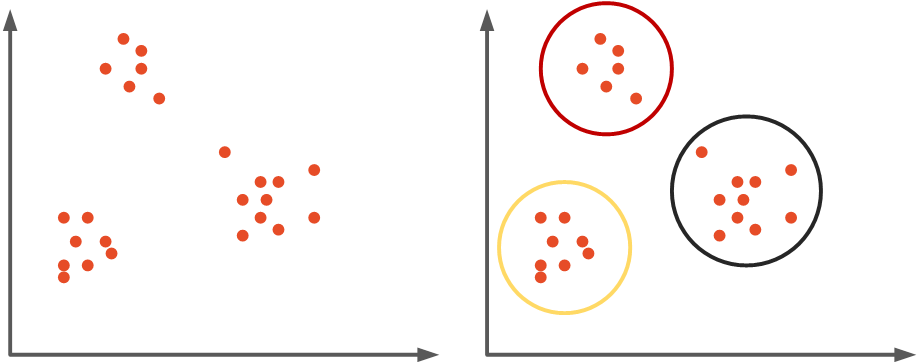There are many clustering algorithms out there and in this article, we cover three of them: K-Means Clustering, Agglomerative Clustering and DBSCAN. As one can imagine, since the dataset is completely unlabeled, deciding which algorithm is optimal for the chosen dataset is much more complicated. Usually, the performance of each algorithm depends on the unknown properties of the probability distribution the dataset was drawn from.

## Dataset and Prerequisites

Data that we use in this article is from PalmerPenguins Dataset. This dataset has been recently introduced as an alternative to the famous Iris dataset. It is created by Dr. Kristen Gorman and the Palmer Station, Antarctica LTER. You can obtain this dataset here, or via Kaggle. This dataset is essentially composed of two datasets, each containing data of 344 penguins. Just like in Iris dataset there are 3 different species of penguins coming from 3 islands in the Palmer Archipelago. Also, these datasets contain culmen dimensions for each species. The culmen is the upper ridge of a bird’s bill. In the simplified penguin’s data, culmen length and depth are renamed as variables culmen_length_mm and culmen_depth_mm.Since this dataset is labeled, we will be able to verify the result of our experiments. However, this is often not the case and validation of clustering algorithm results is often a hard and complicated process.

For the purpose of this article, make sure that you have installed the following Python libraries:

• NumPy – Follow this guide if you need help with installation.
• SciKit Learn – Follow this guide if you need help with installation.
• SciPy – Follow this guide if you need help with installation.
• Yellowbrick – Follow this guide if you need help with installation.

Once installed make sure that you have imported all the necessary modules that are used in this tutorial.

``````import pandas as pd
import numpy as np
import matplotlib.pyplot as plt

from scipy.spatial.distance import cdist
import scipy.cluster.hierarchy as shc
from yellowbrick.cluster import KElbowVisualizer

from sklearn.model_selection import train_test_split
from sklearn.metrics import accuracy_score
from sklearn.preprocessing import StandardScaler
from sklearn.cluster import KMeans, AgglomerativeClustering, DBSCAN``````

Apart from that, it would be good to be at least familiar with the basics of linear algebra, calculus and probability.

## Data Preparation

Ok, let’s prepare data for processing.

``````data = pd.read_csv('./data/penguins_size.csv')

data = data.dropna()
data = data.drop(['sex', 'island', 'flipper_length_mm', 'body_mass_g'], axis=1)

X = data.drop(['species'], axis=1).values
ss = StandardScaler()
X = ss.fit_transform(X)

y = data['species']
spicies = {'Adelie': 0, 'Gentoo': 1, 'Chinstrap': 2}
y = [spicies[item] for item in y]
y = np.array(y) ``````

First, we load it from the .csv file and remove features that we don’t want to consider in this tutorial. Then we split the input data X from the labels y and we scale the input data. The output data is used only for checking out how good our clustering algorithms work. Here is how data looks like when we plot it: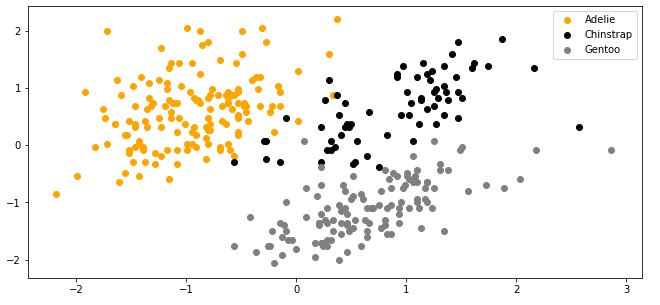## K-Means Clustering

K-Means is one of the most popular clustering algorithms. It is definitely a go-to option when you start experimenting with your unlabeled data. This algorithm groups n data points into K number of clusters, as the name of the algorithm suggests. This algorithm can be split into several stages:

1. In the first stage, we need to set the hyperparameter k. This represents the number of clusters or groups that K-Means Clustering will create once it is done.
2. K random vectors are picked in the feature space. These vectors are called centroids. These vectors are changed during the training process and the goal is to put them into the “center” of each cluster.
3. Distances from each input sample x to each centroid c is calculated using some metric, like Euclidean distance. The closest centroid is assigned to each sample in the dataset. Basically, we create clusters at this stage.
4. For each cluster average feature vector is calculated using samples that are assigned to it. This value is considered as a new centroid of the cluster.
5. Steps 2-4 are repeated for a fixed number of iteration or until the centroids don’t change, whichever comes first.Mathematically speaking each sample x is assigned to a cluster based on: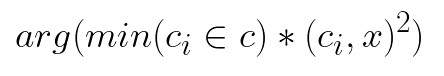where cᵢ is a centroid of a cluster i and D is Euclidean distance calculated using the formula:For finding the new centroid from clustered group of points we use formula: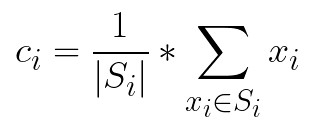As we already mentioned hyperparameter k, ie. the number of clusters, has to be tuned manually. This is the quite tiresome. Later in this tutorial, we consider one technique for selecting the correct number of clusters. However, let’s first implement this simple, yet powerfull algorithm using Python from sratch.

### Python Implementation

This algorithm is implemented within MyKMeansClustering class. The process is quite straight forward, so let’s check out the implementation:

``````class MyKMeansClustering():
def __init__(self, k=2):
self.k = k

def fit(self, X, max_num_iterattions=300, treshold = 0.001):

self.centroids = {}

# Initialize Centroids
for i in range(self.k):
self.centroids[i] = X[i]

for i in range(max_num_iterattions):

# Initialize Clusters
self.clusters = {}
for i in range(self.k):
self.clusters[i] = []

# Euclidian distance for each point
for sample in X:
distances = [np.linalg.norm(sample-self.centroids[centroid]) for centroid in self.centroids]
cluster = distances.index(min(distances))
self.clusters[cluster].append(sample)

# Update centroids
previous_centroids = dict(self.centroids)
for cluster in self.clusters:
self.centroids[cluster] = np.average(self.clusters[cluster], axis=0)

# Check if centroids changed
centroids_changed = True

for centroid in self.centroids:
difference = np.sum((self.centroids[centroid] - previous_centroids[centroid])/
previous_centroids[centroid]*100.0)
if  difference > treshold:
centroids_changed = False

if centroids_changed:
break

def predict(self, sample):
distances = [np.linalg.norm(sample - self.centroids[centroid]) for centroid in self.centroids]
cluster = distances.index(min(distances))
return cluster``````

There are two public methods fit and predict. The number of clusters k is passed through the constructor. The fit method is basically where the magic is happening. First, we initialize centroids by random. Then we calculate the Euclidean distance from each point in the dataset to each centroid. We assign sample to closest centroid, ie. to cluster. Then we update centroids, by taking the average value for each cluster. Finally, we are checking if centroids have changed its value. If they have we stop the process, otherwise we repeat until max_num_iterattions is reached. Also, notice the treshold parameter. Using this parameter we can fine-tune this check. The predict method is used to predict the cluster value for each new sample.

Cool. Let’s try it out on PalmerPenguins dataset:

``````model = MyKMeansClustering(k = 3)
model.fit(X, max_num_iterattions=700, sse_treshold= 0.00001)

clusters = []
for sample in X:
clusters.append(model.predict(sample))

print(accuracy_score(clusters, y))``````
``0.9281437125748503``

This is a bit unorthodox, but in the end, we are checking the accuracy_score score. In normal conditions, we wouldn’t have output information and thus we would not know the result of our actions. However, since this is an educational tutorial, we can see that the K-Means algorithm has done a good job, with an accuracy of ~93%. Let’s print out the output and compare it with the real values:Apparently, there are several missing samples in the middle of the distribution, but overall not bad, not bad at all.

### Using SciKit Learn

Using this algorithm with Sci-Kit is even easier:

``````kmeans = KMeans(n_clusters=3, max_iter = 700, random_state=6)
kclusters = kmeans.fit_predict(X)
print(accuracy_score(kclusters, y))``````
``0.9251497005988024``

Since we are using the predict method on the input data as well, we can utilize the fit_predict method. Accuracy is still ~92% and the visual representation looks almost the same as with our implementation: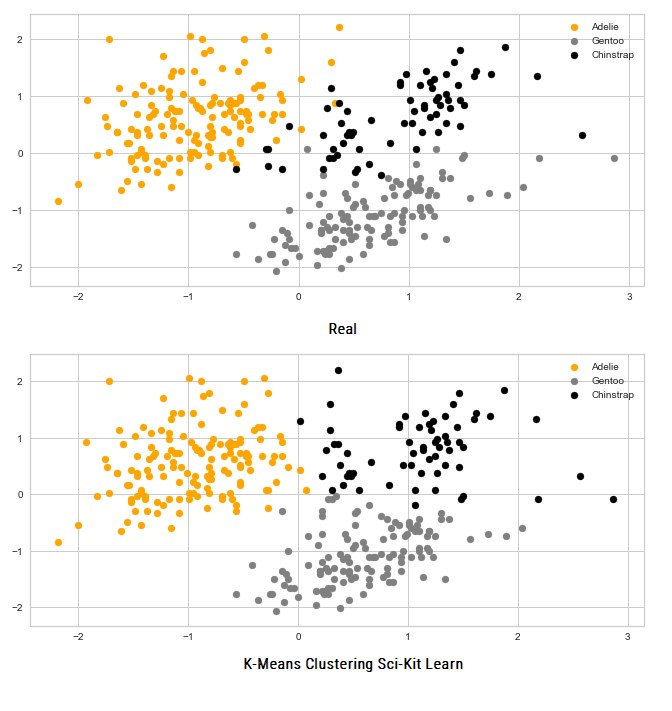Again there are several points in the middle that are not properly clustered, but that data is specifically hard because it represents edge cases.

Things look pretty easy when we know the number of clusters beforehand, but what should we do when we get unlabeled data in the real world. How are we supposed to know the number of clusters? There are many techniques that can help us achieve that. One of the most popular ones is the Elbow method.

### The Elbow Method

We know that we have tree classes in our dataset. However, let’s forget about all that for a second and let’s plot out data using the same color for all classes: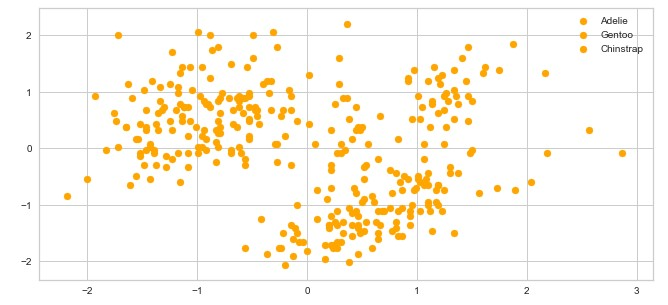How many clusters do you see? We could say 3-ish, but we can not be sure. Plus there is that data in the middle which is super sketchy. As we said one of the most popular methods for determining the number of clusters in the dataset is called the Elbow Method. It can be built on top of two metrics: distortion and inertia. Distortion is calculated as the average of the squared distances (let’s say Euclidean distance) from the cluster centers of the respective clusters. Inertia represents the sum of squared distances of samples to their closest cluster center.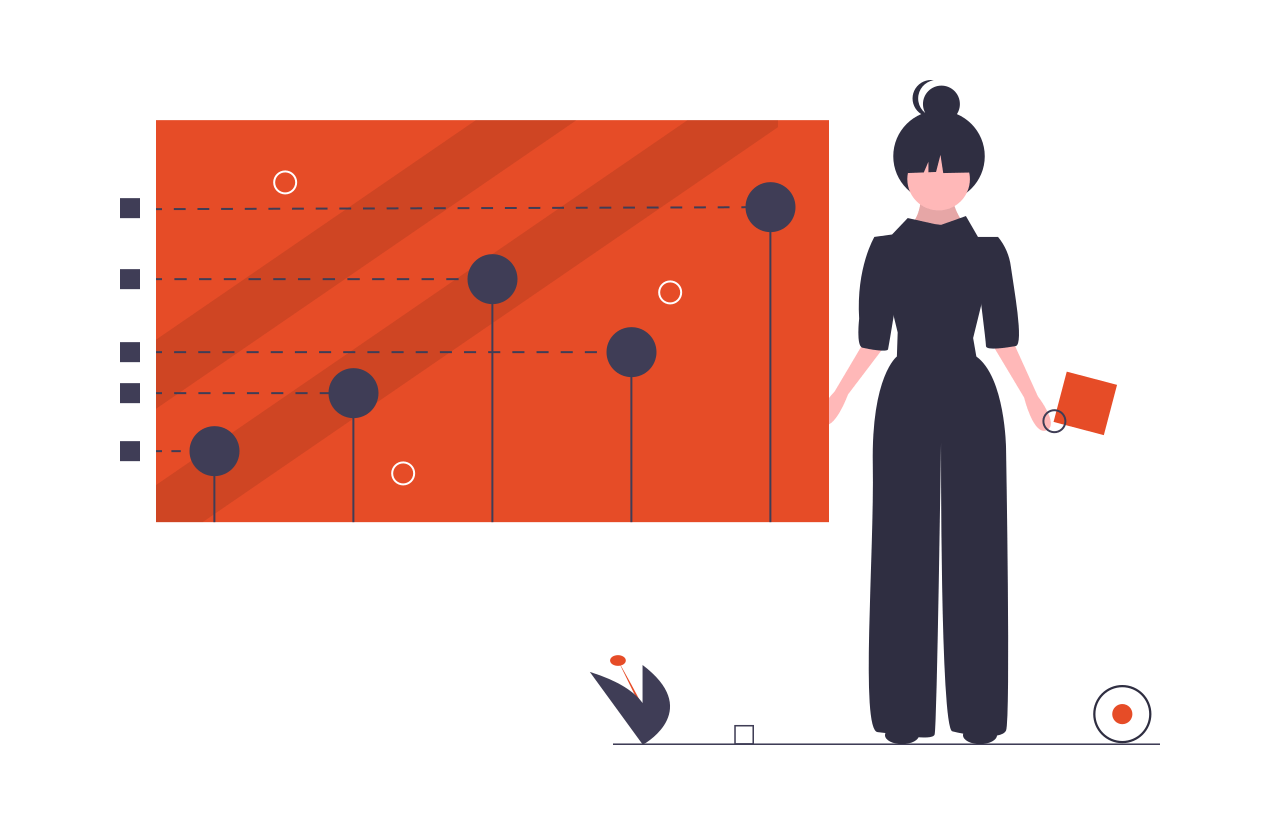What we can do is run our clustering algorithm with a variable number of clusters and calculate distortion and inertia. Then we can plot the results. There we can look for the “elbow” point. This is the point after which the distortion/inertia starts decreasing in a linear fashion as the number of clusters grows. This point tells us the optimal number of clusters. Let’s use calculate both metrics first:

``````distortions = []
inertias = []

for k in range(1,10):
kmeanModel = KMeans(n_clusters=k)
kmeanModel.fit(X)

distortions.append(sum(np.min(cdist(X, kmeanModel.cluster_centers_, 'euclidean'),axis=1)) / X.shape)
inertias.append(kmeanModel.inertia_) ``````

Now let’s plot the distortion value along with the number of clusters: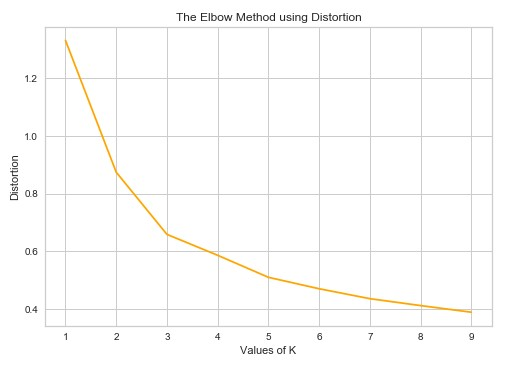From this image we can conclude that after 3 clusters, distortion decreases in a linear fashion, ie. 3 is the optimal number of clusters. How about inertia:We can conclude the same thing from this image as well.

One library can help us with this process – Yellowbrick. This library is built on top of Sci-Kit Learn and it provides a bunch of useful visualizations. Here is how we can get the above result using it:

``````model = KMeans()
visualizer = KElbowVisualizer(model, k=(1,10))

visualizer.fit(X)
visualizer.show()``````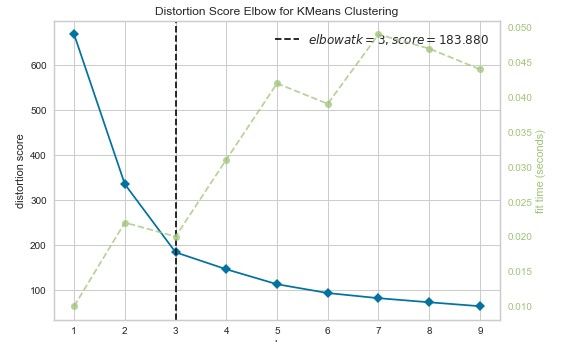Again this visualizer used distortion as a metric. It can be useful when we are not quite sure when our metric starts to be linear.

## Agglomerative Clustering

As we can see one of the biggest challenges of working with K-Means is that we need to determine the number of clusters beforehand. Another challenge is that K-Means tries to make clusters of the same size. These challenges can be addressed with other algorithms like Hierarchical Clustering. In general, every Hierarchical Clustering method starts by putting all samples into separate single-sample clusters. Then based on some similarity metrics, samples or clusters are merged together until the point when all samples are put into a single cluster. This means that we are building the hierarchy of clusters, hence the name. In this article, we explore Agglomerative Clustering which is one specific type of Hierarchical Clustering. The metric that it uses for merging clusters is the distance, ie. it merges the closest pair of clusters, based on the distance among centroids and repeats this step until only a single cluster is left. For this purpose, the proximity matrix is used. This matrix stores the distances between each point.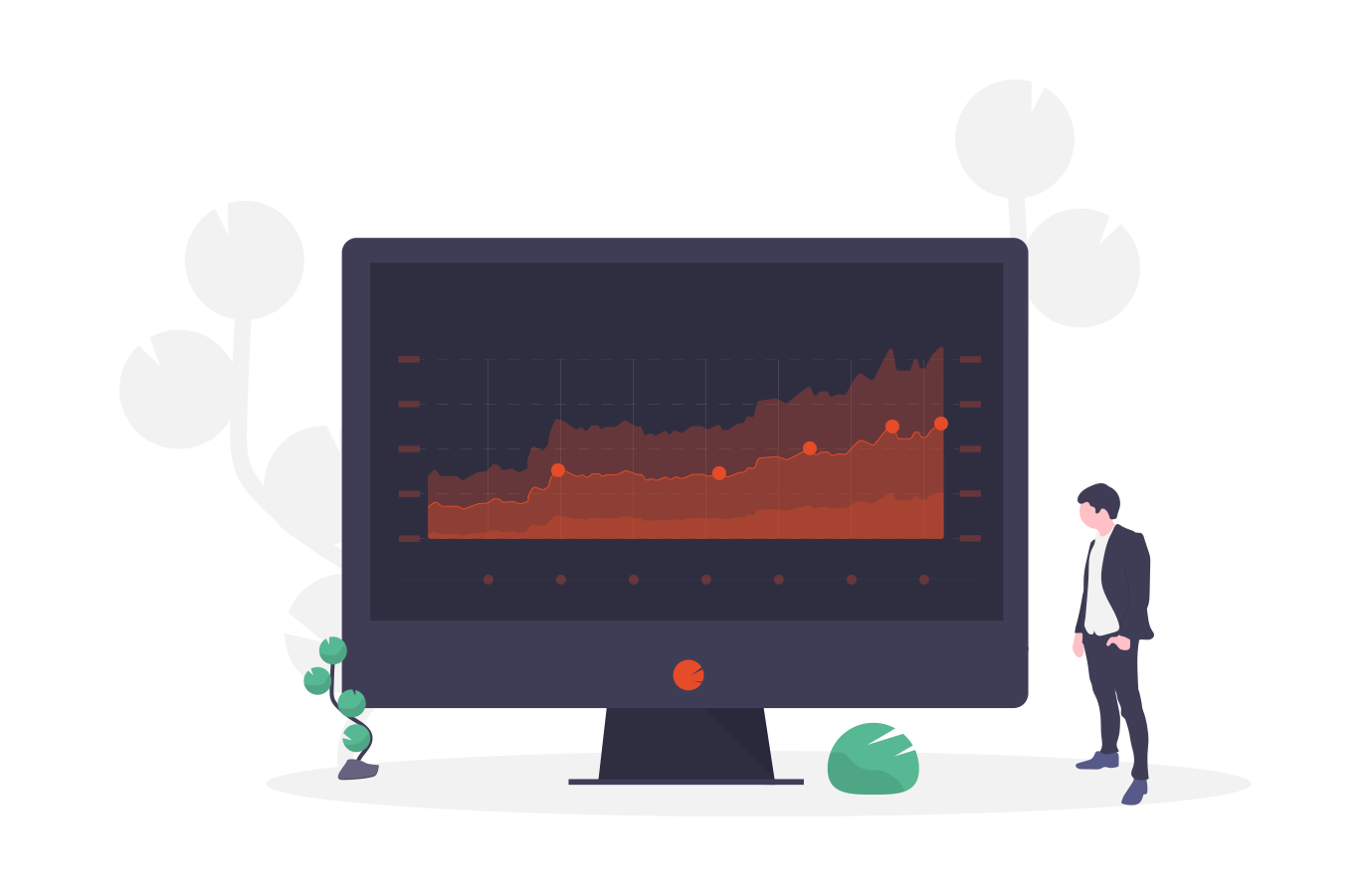Let’s break this into steps:

1. Every point is stored in its own cluster
2. The proximity Matrix is calculated
3. Closest points are detected and merged. They are the cluster and centroid is calculated.
4. The proximity matrix is updated using the centroid of the created cluster.
5. Steps 3 and 4 are repeated until one cluster is created.

At this moment you might ask yourself, how does this help us with deciding the number of clusters? To do so, we utilize an awesome concept – Dendrogram.

### Dendrogram

This is a tree diagram that records all merges that happened during the training process. So, every time we merge two points or clusters, this is stored in the dendrogram. Here is how we can create it using SciPy:

``````plt.figure(figsize=(10, 7))
plt.title("Dendrograms")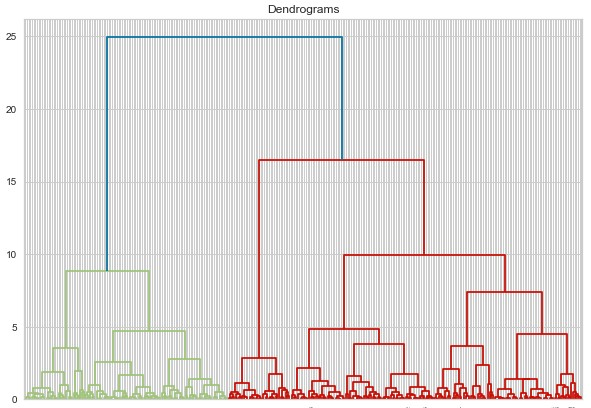What are we looking at? Well, on the x-axis we have all points in the dataset, while on the y-axis we have distances between those points. Every time points or clusters are merged this is represented with a horizontal line. The vertical line represents the distances between merged points/clusters. Longer vertical lines in the dendrogram indicate that the distance between clusters is bigger. In the next step, we need to set a threshold distance and draw a horizontal line in this image. In general, we try to set the threshold in such a way that it cuts the tallest vertical line. In our example, we set it to 15. Here is how that is done:

``````plt.figure(figsize=(10, 7))
plt.title("Dendrograms")
plt.axhline(y=15, color='r', linestyle='--')``````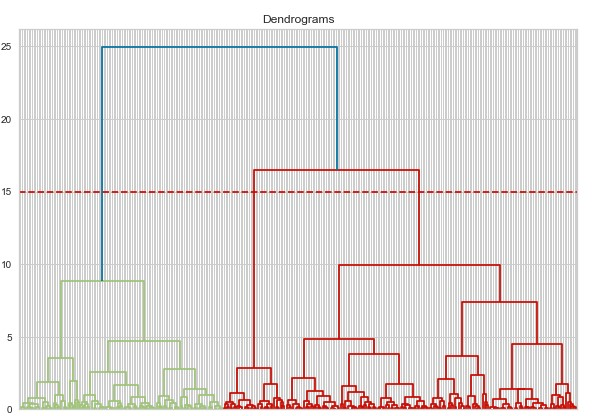The number of clusters is determined by the number of vertical lines that are being intersected by the threshold line. As we can see the number of clusters in our example is 3.

### Using Sci-Kit Learn

Alright, now we know how Agglomerative Clustering works behind the curtains. Let’s see how we can use it with Sci-Kit Learn:

``````aglo = AgglomerativeClustering(n_clusters=3)
aclusters = aglo.fit_predict(X)
print(accuracy_score(aclusters, y))``````
``0.9011976047904192``

The accuracy is a bit smaller than when we were using K-Means Clustering. Let’s plot data and see how it compares with the real situation:We can see that cluster of ‘Chinstrap’ class is much smaller than it is in reallity and that is where the lower accuracy comes from.

## DBSCAN

Unlike K-Means and Hierarchical Clustering, which are centroid-based algorithms, DBSCAN is a density-based algorithm. Effectively, this means that you don’t need to determine how many clusters do you need. We saw this at Hierarchical clustering, but DBSCAN takes it to another level. Instead of defining hyperparameter k, we define two hyperparameters for distance ε and the number of samples per cluster – n. Let’s break it into steps and it will be more clear:

1. First, we assign a random sample x to the first cluster.
2. We count how many samples have a distance from the sample x that is less or equal to ε. If the number of such samples is greater or equal to n, we add them in the cluster.
3. We observe each new member of the cluster and perform step 2 for them too, ie. we calculate the number of samples within the ε area of the sample and if that number is larger then n, we add them to the cluster. We repeat this process recursively until there are no more samples left to put in it.
4. Steps from 1 to 3 for a new random unclustered sample.
5. The process is repeated like this until all samples are either clustered or marked as outliers.The main advantage of this approach is that clusters have different and random shapes. Centroid-based algorithms always create clusters that have the shape of a hypersphere. That is why DBSCAN can be specifically useful for some data. Of course, the main problem is choosing optimal values for ε and n. This problem is optimized with a variation of this algorithm called HDBSCAN, ie. high-performance DBSCAN. This algorithm eliminates the use of the ε hyperparameter, however, this algorithm is out of the scope of this tutorial.

### Using Sci-Kit Learn

Ok, let’s run PalmerPenguins data through DBSCAN with Sci-Kit Learn implementation:

``````dbscan = DBSCAN(eps = 0.89, min_samples=74)
dbclusters = dbscan.fit_predict(X)
print(accuracy_score(dbclusters, y))``````
``0.7694610778443114``

With this algorithm, we got the worst accuracy. However, keep in mind that this doesn’t mean that this is a bad algorithm, just that it is not suitable for this data, or we need to further experiment and use better hyperparameters. When we plot the data and compare it with the real situation: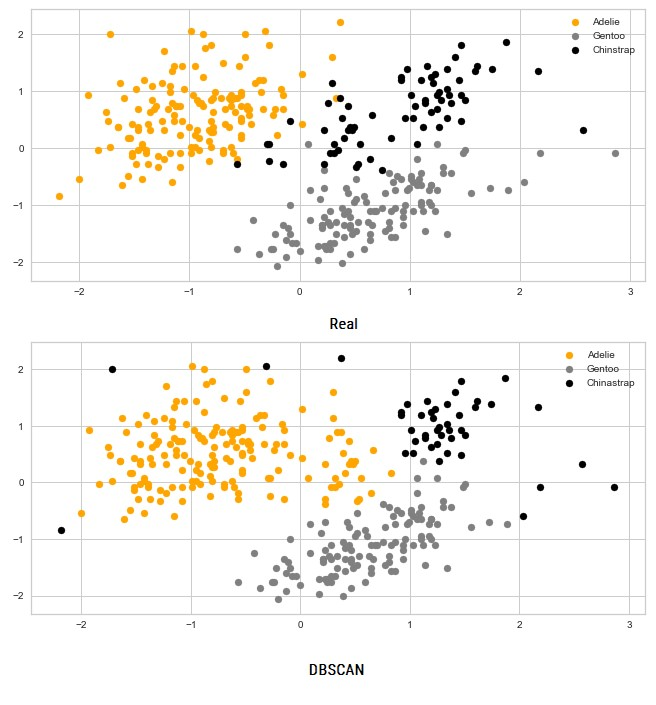Interesting results indeed. Especially if we take into consideration that this is done based on the density.

## Conclusion

In this article we had a chance to explore how we can utilize unsupervised learning for clustering problems. We observed three algorithms K-Means CLustering, Hierarchical Clustering and DBSCAN. We applied them to PalmerPenguins dataset and saw some really interesting results. Also, we had a chance to see how powerfull unsupervised learning can be.#### Nikola M. Zivkovic

CAIO at Rubik's Code

Nikola M. Zivkovic a CAIO at Rubik’s Code and the author of book “Deep Learning for Programmers“. He is loves knowledge sharing, and he is experienced speaker. You can find him speaking at meetups, conferences and as a guest lecturer at the University of Novi Sad.

Rubik’s Code is a boutique data science and software service company with more than 10 years of experience in Machine Learning, Artificial Intelligence & Software development. Check out the services we provide.

Ultimate Guide to Machine Learning with Python

Everything from Python basics to the deployment of Machine Learning algorithms to production in one place.

Become a Machine Learning Superhero TODAY!